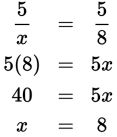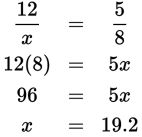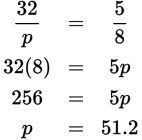# SAT Math Multiple Choice Question 966: Answer and Explanation

### Test Information

Question: 966

2. A triangle with side lengths of 5, 12, and 15 centimeters is similar to another triangle.The longest side of the other triangle has length 24 centimeters. What is the perimeter,in centimeters, of the larger triangle?

• A. 38.4
• B. 44
• C. 51.2
• D. 58

Explanation:

C

Difficulty: Medium

Category: Additional Topics in Math / Geometry

Strategic Advice: Corresponding sides of similar triangles are in proportion to each other. Because you know the length of the longest side for both triangles, you can set up and solve a proportion to find the lengths of the other two sides of the larger triangle.

Getting to the Answer: The longest sides are in the ratio 15:24, which simplifies to 5:8. Use this in your proportions:

 Shortest side to longest side Medium side to longest sideThe perimeter of the larger triangle is the sum of the side lengths, 8 + 19.2 + 24 = 51.2 cm, which is (C).

Note: There is a shortcut for this type of question if you happen to remember that the perimeters of similar triangles are also in the same proportion as the sides. The perimeter of the smaller triangle is 5 + 12 + 15 = 32. You could set up and solve the same type of proportion here: